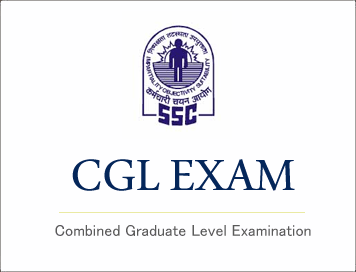# SSC CGL (Tier - 1) Online Exam Paper - 2016 "held on 4 September 2016" Morning Shift (Quantitative Aptitude)## SSC CGL (Tier - 1) Online Exam Paper - 2016 "held on 4 September 2016" Morning Shift (Quantitative Aptitude)

EXAM DATE : - 4 September-2016
EXAM START TIME : 10:00:00
EXAM NAME : SSC Examination 2016

Question 51.X can do a piece of work in 'p' days and Y can do the same work in 'q' days . Then the number of days in which X and Y can together do that work isQuestion 52.A shopkeeper marks his goods 40% above the cost price and allows a discount of 25% on it. His gain % is

Options:
1) 5%
2) 10%
3) 15%
4) 20%

Question 53.The ratio of the ages of two boys is 3:4. After 3 years, the ratio will be 4:5.The ratio of their ages after 21 years will be

Options:
1) 14:17
2) 17:19
3) 11:12
4) 10:11

Question 54.The cost price of 25 books is equal to the selling price of 20 books. The profit percent is

Options:
1) 20%
2) 22%
3) 24%
4) 25%

Question 55.One number is 25% of another number. The larger number is 12 more than the smaller. The large number is

Options:
1) 48
2) 16
3) 4
4) 12

Question 56.A train 500 m long, running at a uniform speed, passes a station in 35 sec. If the length of the platform is 221 m, the speed of the train in km/hr is

Options:
1) 721/35
2) 74.16
3) 24.76
4) 78.54

Question 57.If the simple interest on Rs. 400 for 10 years is Rs. 280, then rate of interest per annum is

Options:
1) 7%
2) 7 ½ %
3) 7 ¼ %
4) 8 ½ %

Question 58.Options:
1) -1
2) 1
3) 0
4) 1/2

Question 59.Options:
1) 1/5
2) 1/6
3) 5
4) 6

Question 60.G and AD are respectively the centroid and median of the triangle ΔABC.The ratio AG:AD is

Options:
1) 3:2
2) 2:3
3) 2:1
4) 1:2

Question 61.A point P lying inside a triangle is equidistant from the vertices of the triangle. Then the triangle has P as its

Options:
1) Centroid
2) Incentre
3) Orthocentre
4) Circumcentre

Question 62.If sinθ + cosθ = 1, then the sinθ cosθ is equal to

Options:
1) 0
2) 1
3) ½
4) -½

Question 63.If 7 times the seventh term of an Arithmetic Progression (AP) is equal to 11 times its eleventh term, then the 18th term of the AP will be

Options:
1) 1
2) 0
3) 2
4) -1

Question 64.The average age of eight teachers in a school is 40 years. A teacher among them died at the age of 55 years whereas another teacher whose age was 39 years joins them. The average age of the teachers in the school now is (in years)

Options:
1) 35
2) 36
3) 38
4) 39

Question 65.Options:
1) 0
2) 1
3) 2
4) 3

Question 66.Options:
1) 3/4
2) 4/5
3) 5/8
4) 7/8

Question 67.Two equal circles of radius 3 cm each and distance between their centres is 10 cm. The length of one of their transverse common tangent is

Options:
1) 7 cm
2) 9 cm
3) 10 cm
4) 8 cm

Correct Answer: 8 cm

Question 68.Options:
1) 90o
2) 45 o
3) 60 o
4) 75 o

Question 69.Options:
1) 4/3
2) 3/4
3) 5/3
4) 3/5

Question 70.A sphere has the same curved surface area as a cone of vertical height 40 cm and radius 30 cm. The radius of the sphere is

Options:
1) 5√5 cm
2) 5√3 cm
3) 5√15 cm
4) 5√10 cm

Correct Answer: 5√15 cm

Question 71.The angle of elevation of the top of a tower from a point A on the ground is 30˚. On moving a distance of 20 metres towards the foot of the tower to a point B, the angle of elevation increases to 60˚. The height of the tower in metres is

Options:
1) √3
2) 5√3
3) 10√3
4) 20√3

Question 72.Given here is a pie chart of the cost of gold in 2010, 2011, 2012 and 2013. Study the chart and answer the following questionsIf the price of gold in 2013 is Rs. 31,500 per 10 gram, then the price of gold in 2011 per 10 gram is

Options:
1) Rs.17000
2) Rs.17500
3) Rs.18000
4) Rs.18500

Question 73.Given here is a pie chart of the cost of gold in 2010, 2011, 2012 and 2013. Study the chart and answer the following questionsThe ratio of the price of gold in the two years 2010 and 2013 is

Options:
1) 1:2
2) 1:3
3) 1:4
4) 1:5

Question 74.Given here is a pie chart of the cost of gold in 2010, 2011, 2012 and 2013. Study the chart and answer the following questionsThe percentage of increase in the price of gold from the year 2011 to 2013 is

Options:
1) 50%
2) 60%
3) 70%
4) 80%

Question 75.Given here is a pie chart of the cost of gold in 2010, 2011, 2012 and 2013. Study the chart and answer the following questionsThe ratio of percentage of increase in price of gold from 2011 to 2012 and 2012 to 2013 is

Options:
1) 6:5
2) 7:5
3) 8:5
4) 9:5